Courses

# Stresses in the Ground Civil Engineering (CE) Notes | EduRev

## Civil Engineering (CE) : Stresses in the Ground Civil Engineering (CE) Notes | EduRev

The document Stresses in the Ground Civil Engineering (CE) Notes | EduRev is a part of the Civil Engineering (CE) Course Soil Mechanics.
All you need of Civil Engineering (CE) at this link: Civil Engineering (CE)

Stresses in the Ground

Total Stress
When a load is applied to soil, it is carried by the solid grains and the water in the pores. The total vertical stress acting at a point below the ground surface is due to the weight of everything that lies above, including soil, water, and surface loading. Total stress thus increases with depth and with unit weight.

Vertical total stress at depth z, sv = g.Z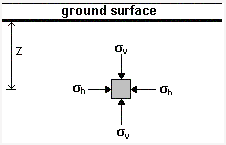Below a water body, the total stress is the sum of the weight of the soil up to the surface and the weight of water above this. sv = g.Z + gw.Zw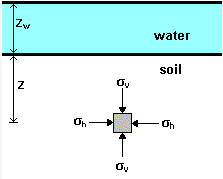The total stress may also be denoted by sz or just s. It varies with changes in water level and with excavation

Pore Water Pressure
The pressure of water in the pores of the soil is called pore water pressure (u). The magnitude of pore water pressure depends on:

• the depth below the water table.
• the conditions of seepage flow.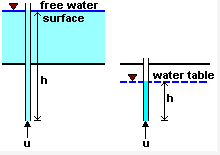Under hydrostatic conditions, no water flow takes place, and the pore pressure at a given point is given by u = gw.h

where h = depth below water table or overlying water surface

It is convenient to think of pore water pressure as the pressure exerted by a column of water in an imaginary standpipe inserted at the given point.

The natural level of ground water is called the water table or the phreatic surface. Under conditions of no seepage flow, the water table is horizontal. The magnitude of the pore water pressure at the water table is zero. Below the water table, pore water pressures are positive.

Principle of Effective Stress

The principle of effective stress was enunciated by Karl Terzaghi in the year 1936. This principle is valid only for saturated soils, and consists of two parts:

1. At any point in a soil mass, the effective stress (represented by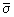or s) is related to total stress (s) and pore water pressure (u) as= s - u

Both the total stress and pore water pressure can be measured at any point.

2. All measurable effects of a change of stress, such as compression and a change of shearing resistance, are exclusively due to changes in effective stress.

Compression =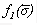Shear Strength =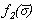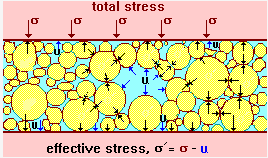In a saturated soil system, as the voids are completely filled with water, the pore water pressure acts equally in all directions.

The effective stress is not the exact contact stress between particles but the distribution of load carried by the soil particles over the area considered. It cannot be measured and can only be computed.

If the total stress is increased due to additional load applied to the soil, the pore water pressure initially increases to counteract the additional stress. This increase in pressure within the pores might cause water to drain out of the soil mass, and the load is transferred to the solid grains. This will lead to the increase of effective stress.

Offer running on EduRev: Apply code STAYHOME200 to get INR 200 off on our premium plan EduRev Infinity!

,

,

,

,

,

,

,

,

,

,

,

,

,

,

,

,

,

,

,

,

,

;